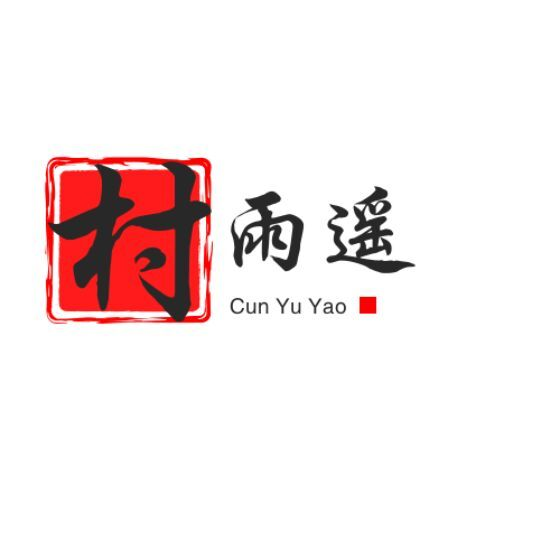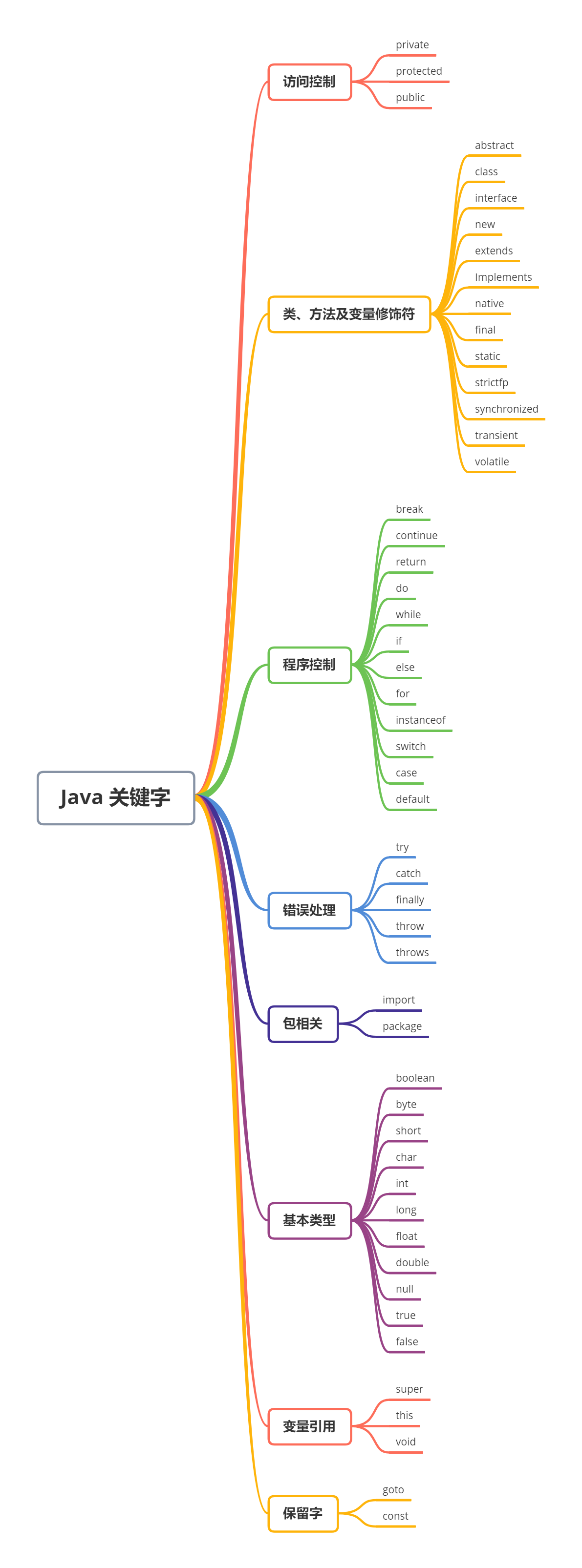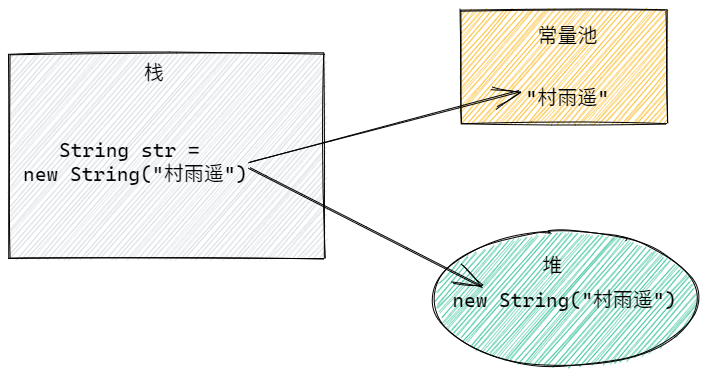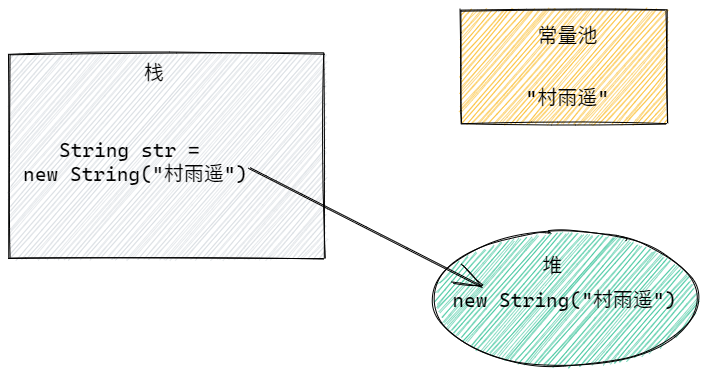# 【Java 从入坑到放弃】No 3. 变量与数据类型1. 变量

2. 数据类型

3. 变量作用域

4. 常量

## 变量

### 什么是变量？

• 基本类型的变量

• 引用类型的变量

// 基本类型的变量int id = 1;// 引用类型的变量String name = "村雨遥";

idname 则是标识符，也就是我们所说的 变量

= 则是赋值操作符，而 1 则是基本类型的值，村雨遥 则是引用类型的值；

### 变量的特点

public class Main {    public static void main(String[] args) {    // 定义int类型变量id，并赋予初始值1        int id = 1;     // 打印该变量的值，观察是否为1        System.out.println(id);      // 重新赋值为2        id = 2;         // 打印该变量的值，观察是否为2        System.out.println(id);     }}

### 变量命名规则

1. 强制：变量命名只能使用 字母（大小写均可）、数字、$、_ 2. 强制：变量名不能使用关键字（就是 Java 中内置的一些关键字，如 int、for、long…）； 3. 强制：变量第一个字符不能使用数字，只能用字母、$_

4. 更多命名规则推荐参考阿里巴巴推出的《Java 开发手册》，下载链接：https://github.com/cunyu1943/amazing-books

### 常见关键字

• 访问控制类

• 类、方法及变量修饰符类

• 程序控制类

• 错误处理

• 包相关

• 基本类型

• 变量引用

• 保留字## 数据类型

### 基本数据类型

Java 中，共有 8 中基本数据类型，由 Java 语言预定好的，每个数据类型都属于关键字，而且每种基本变量都有其对应的封装类，这 8 中基本数据类型分别是：

• 整型（4 种）

• 浮点型（2 种）

• 字符型（1 种）

• 布尔型（1 种）

1. boolean 一般用 1 bit 来存储，但是具体大小并未规定，JVM 在编译期将 boolean 类型转换为 int，此时 1 代表 true0 代表 false。此外，JVM 还指出 boolean 数组，但底层是通过 byte 数组来实现;

2. 使用 long 类型时，需要在后边加上 L，否则将其作为整型解析，可能会导致越界；

3. 浮点数如果没有明确指定 float 还是 double，统一按 double 处理；

4. char 是用 单引号 '' 将内容括起来，只能存放一个字符，相当于一个整型值（ASCII 值），能够参加表达式运算；而 String 是用 双引号 "" 将内容括起来，代表的是一个地址值；

5. Java 在内存中是采用 Unicode 表示，所以无论是一个中文字符还是英文字符，都能用 char 来表示；

float score = 89.0f;int age = 26;

### 引用数据类型

#### 常见引用数据类型

// 初始化一个对象Pet dog = new Pet();// 初始化一个数组int[] arr = new int;

#### String

String 不同于 char，它属于引用类型，而 char 属于基本数据类型。用双引号 "" 括起来表示字符串，一个字符串能够保存 0 个到任意个字符，它一旦创建就不能被改变。

Java 编译器中，对于字符串和其他数据类型之间，可以使用 + 进行连接，编译器会自动将其他数据类型自动转换为字符串，然后再进行连接；

String 既然是不可变，那有什么优点呢？

1. 用于缓存 hash

1. 字符串常量池（String Pool）的需要

1. 安全性

1. 线程安全

String 的不可变性让它天生 具备线程安全，能够在多个线程中方便使用而不用考虑线程安全问题。

String、StringBuilder、StringBuffer 对比，该如何选择？

1. String Pool 中不存在 “xxx”1. String Pool 中存在 “xxx”### 数据类型转换

int a = 110;long b = 113;// 低精度转高精度，由于 long 的范围比 int 大，所以可以自动转b = a;// 高精度住哪低精度，由于 long 的范围比 int 大，所以需要强制转a = (int)b;

#### 隐式转换（自动类型转换）

1. 两种数据类型彼此兼容；

2. 目标数据类型的取值范围大于源数据类型；

• 数值类型byte -> short -> int -> long -> float -> double

• 字符类型转整型char -> int

#### 显式转换（强制类型转换）

(type) variableName;

int num = 3;double ans = 5.0;// 要将 double 类型的值赋值给 int，则需要强制转换num = (int)ans;

**注意：**强制转换可能会导致精度丢失，所以一般情况下尽量能不用就不用。

#### 常见数据类型转换方法

1. 字符串与其他类型之间的转换

• 其他类型 -> 字符串

1. 调用类的串转换方法：X.toString()

2. 自动转换："" + X

3. 利用 String 的方法：String.valueOf(X)

// 方法 1String str1 = Integer.toString(int num);String str2 = Long.toString(long num);String str3 = Float.toString(flaot num);String str4 = Double.toString(double num);// 方法 2String str = "" + num ; // num 是 int、long、float、double 类型// 方法 3String str1 = String.valueOf(int num);String str2 = String.valueOf(long num);String str3 = String.valueOf(float num);String str4 = String.valueOf(double num);

• 字符串 - > 其他类型

1. 调用 parseXXX 方法，比如 parseLong、parseFloat、parseDouble...

2. 先调用 valueOf()，方法，然后再调用 xxxValue() 方法；

// 方法 1int num1 = Integer.parseInt(String str);Long num2 = Long.parseLong(String str);Float num3 = Float.parseFloat(String str);Double num4 = Double.parseDouble(String str);// 方法 2int num1 = Integer.valueOf(String str).intValue();Long num2 = Long.valueOf(String str).longValue();Float num1 = Float.valueOf(String str).floatValue();Double num1 = Double.valueOf(String str).doubleValue();

1. int、float、double 之间的转换

• float -> double

float num = 1.0f;Float num1 = new Float(num);double num2 = num1.doubleValue();

• double -> float

double num = 100.0;float num1 = (float)num;

• double -> int

double num = 100.0;Double num1 = new Double(num);int num2 = num1.intValue();

• int -> double

int num = 200;double num1 = num;

## 变量作用域

• 成员变量：定义在方法体和语句块外，不属于任何一个方法，能在整个类中起作用；

• 局部变量：定义在方法或方法体中的变量，作用域是其所在的代码块；

### 成员变量

public class Person {    // 成员变量，全局变量    String name;     // 成员变量，全局变量    int age;     // 成员变量，静态变量    public static final String wechatPublic = "公众号：村雨遥";     // 成员变量，静态变量    public static final String website = "http://cunyu1943.site"; }

### 局部变量

• 形参

public class Main {    // 方法中的参数    public static void func(int num) {        System.out.println("num = " + num);    }    public static void main(String[] args) {        func(3);    }}

• 方法内定义

public class Main {    public static void main(String[] args) {        int num = 10;        if (num > 5) {            // 声明一个 int 类型的局部变量            int tmp = 5;             System.out.println("tmp = " + tmp);            System.out.println("num = " + num);        }        System.out.println("num = " + num);    }}

• 代码块定义

public class Main {    public static void func() {        try {            System.out.println("Hello!Exception!");        } catch (Exception e) { // 异常处理块，参数为 Exception 类型            e.printStackTrace();        }    }    public static void main(String[] args) {        func();    }}

## 常量

### 分类

Java 中使用 final 关键字来声明常量，其语法格式如下：

final 数据类型 常量名 = 常量初始值;

public class Main{    public static void main(String[] args){        // 声明一个常量并赋值        final int num = 1024;                // 再次赋值，将导致编译错误        num = 1943;                // 声明一个常量但不赋值        final int id;        // 因为声明时未赋值，所以可以进程初次赋值        id = 1;        // 常量已经赋值过了，再次赋值将导致编译错误        id = 2;    }}

• 静态常量final 之前用 public staic 修饰，表示该常量的作用域是全局的，我们不用创建对象就能够访问它。

• 成员常量：类似于成员变量，但是最大的不同在于它不能被修改。

• 局部常量：作用类似于局部变量，不同之处也在于不能修改。

public class Main{    // 静态变量    public static final dobule PI = 3.14;        // 成员常量    final int num = 1024;        public static void main(String[] args){        // 局部变量        final long count = 1000;    }}

PS：final 修饰变量后，该变量则变为常量。而 final 也还可以用来修饰类和方法，修饰方法时，表示这个方法不能被重写（但可以重载）；修饰类时，则表明该类无法被继承。这些东西这时候你可能会觉得很陌生，不过等我们后续学习了面向对象之后，你就会发现其实很简单。

## 总结## 评论